# Difference between revisions of "10-327/Homework Assignment 4"

## Contents

### Doing

Solve and submit problems 1-3 and 8-10 Munkres' book, pages 157-158.

### Due date

This assignment is due at the end of class on Monday, October 25, 2010.

### Suggestions for Good Deeds

Annotate our Monday videos (starting with ) in a manner similar to (say) , and/or add links to the blackboard shots, in a manner similar to . Also, make constructive suggestions to me, Dror and / or the videographer, Qian (Sindy) Li, on how to improve the videos and / or the software used to display them. Note that "constructive" means also, "something that can be implemented relatively easily in the real world, given limited resources".

 Dror's notes above / Student's notes below

### Questions

1. Hi, quick question. I am wondering if the term test will cover the material on this assignment, or only the material before the assignment. Thanks! Jason.
• The term test will cover everything including Monday October 25 and including this assignment. Drorbn 13:42, 23 October 2010 (EDT)
2. In EXAMPLE 7 on page 151 Munkres claims that Rn~ is ['clearly' :)] homeomorphic to Rn: where Rn~ consists of all sequences x=(x1,x2,x3,...) with xi=0 for i>n, and Rn consists of all sequences x=(x1,x2,...xn). Why are they homeomorphic ?? Thank you kindly. Oliviu.

RE: 2) Let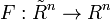$F :\tilde R^n \rightarrow R^n$ be defined as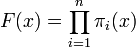$F(x)= \prod_{i=1}^{n} \pi_i (x)$ and let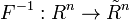$F^{-1} : R^n \rightarrow \tilde R^n$ be defined as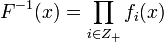$F^{-1}(x)= \prod_{i \in Z_+} f_i (x)$ where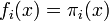$f_i (x) = \pi_i (x)$ if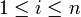$1 \le i \le n$ and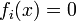$f_i(x)=0$ otherwise. Then both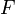$F$ and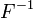$F^{-1}$ are continuous, because we are working in the product topology and the component functions, namely the projection function and constant function are continuous. Also$F$ is a bijection because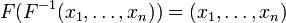$F(F^{-1}(x_1, \ldots, x_n))=(x_1, \ldots, x_n)$ and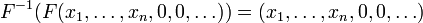$F^{-1}(F(x_1, \ldots, x_n, 0,0, \ldots))=(x_1, \ldots, x_n, 0,0, \ldots)$, i.e$F$ has a left and right inverse. So$F$ is a homeomorphism between the two spaces. Quick question is there a nicer way of writing math than using the math tag? Ian 16:03, 22 October 2010 (EDT)

• Hi Ian I don't believe what you have said.[/itex]\tilde R^n[/itex] has the subspace topology from [/itex]R^\omega[/itex] where we indeed put product topology on it. This is not the same as [/itex]\tilde R^n[/itex] has the product topology. -Kai
• Kai I don't understand what you mean by not the same can you clarify? Do you mean they are not homeomorphic, i.e. there is something wrong with the functions I provided? Ian 17:47, 27 October 2010 (EDT)
• Hi Kai I see what you are trying to say now. Theorem 19.3 suggests they are they same. Ian 19:49, 27 October 2010 (EDT)

3)Question. Suppose we have a function f going from topological space X to Y which is not onto and a function g going from Y to Z. Could I still define the composition of f and g? i.e. g circle f? -Kai Xwbdsb 19:19, 22 October 2010 (EDT)

• If I understand your question, I don't see why not...think about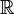$\mathbb{R}$ for example.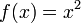$f(x)=x^2$ is not onto, then let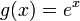$g(x)=e^x$ then g compose f is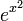$e^{x^2}$ - John
• I agree but look at munkre's page 17 last sentence. Note that g compose with f is defined only when the range of f equals the domain of g. So I just want to confirm with Dror if there is something wrong here.
• Touche, I see your point...that is strange - John

4)Question about the proof for [0,1] being connected. A few details are omitted. why would a closed subset of [0,1] contain its supremum? Also why [0,g_0] being a subset of A follows automatically after we showed that g_0 is in A? -Kai

• 1. Suppose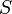$S$ is closed in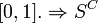$[0,1]. \Rightarrow S^C$ is open. If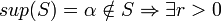$sup(S)=\alpha \notin S \Rightarrow \exists r>0$ s.t.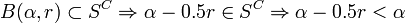$B(\alpha, r) \subset S^C \Rightarrow \alpha - 0.5r \in S^C \Rightarrow \alpha - 0.5r < \alpha$ is an upper bound for S.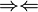$\Rightarrow \Leftarrow$
• 2. Recall that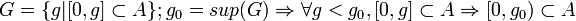$G = \{g | [0,g] \subset A\}; g_0 = sup(G) \Rightarrow \forall g < g_0, [0,g] \subset A \Rightarrow [0, g_0) \subset A$. So, if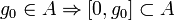$g_0 \in A \Rightarrow [0,g_0] \subset A$. -Frank Fzhao 23:50, 22 October 2010 (EDT)
• Thanks Frank. But I don't think your solution is convincing enough. \alpha - 0.5r is indeed not in S but why can you say it is an upper bound for S? Remember S could be rather complicated set all you know is that it is closed.

for 2 why is \Rightarrow \forall g < g_0, [0,g]? even if g_0 is sup(G) that does not mean anything less than g_0 would be in G. Consider [0,1] union {3}.

• Well, for the first question, not only is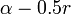$\alpha - 0.5r$ not in S, but neither is anything in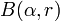$B(\alpha,r)$, since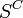$S^C$ is open. There can be no elements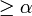$\geq \alpha$ in S because it's the supremum. Recall also we're working in the Reals.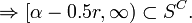$\Rightarrow [\alpha - 0.5r, \infty) \subset S^C.$
• For the second question, notice that the supremum is the least upper bound (of G), so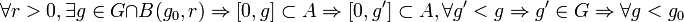$\forall r > 0, \exists g \in G \cap B(g_0,r) \Rightarrow [0,g] \subset A \Rightarrow [0,g'] \subset A, \forall g' < g \Rightarrow g' \in G \Rightarrow \forall g < g_0$, take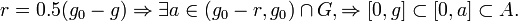$r = 0.5(g_0 - g) \Rightarrow \exists a \in (g_0 - r, g_0) \cap G, \Rightarrow [0,g] \subset [0,a] \subset A.$

Specifically, in your counterexample, if 3 is in G, then anything less than 3 is also in G by construction of G.

• Perhaps (I'm guessing here) you might have found supremum to be a confusing notion. If this is the case, have no fear, there's a chapter on supremum in Spivak's book Calculus. You can probably find one in the math library. - Frank Fzhao 09:58, 23 October 2010 (EDT)
• Question 1(b) on HW4. x stands for a point in S^1 but what does it mean by -x? -Kai
•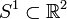$S^1\subset{\mathbb R}^2$, and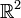${\mathbb R}^2$ is a vector space. Drorbn 13:42, 23 October 2010 (EDT)
• Question. During the proof of A \subset B \subset of A closure when they are all subspaces of X. If A is connected and so is B. I think there is loss of generality. We should prove any non-empty clopen set is B. But we are only proving those clopen sets whose intersection with A is non-empty are B. That is not enough because we could also have non-empty clopen set in B which does not intersect A. -Kai
• If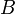$B$ doesn't intersect$A$ it's complement will. Drorbn 13:42, 23 October 2010 (EDT)
• Question. Consider the usual basis for infinite product topology that we put on an infinite cartesian product of topological spaces. The basis is just the topology generated minus the empty set right? Xwbdsb 23:23, 23 October 2010 (EDT)
• No. In an infinite (or even finite) product there are open sets which are unions of cylinders but are not themselves cylinders. A ball in the plane is a union of boxes but it is not a box. Another example is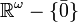${\mathbb R}^\omega-\{\bar{0}\}$. Drorbn 11:45, 24 October 2010 (EDT)
• Another question. We proved before if we have a collection of metrizable topological spaces then if we put cylinder topology on the infinite cartesian product then the resulting topological space is going to be metrizable. Then why isnt R^R with cylinder topology metrizable a contradiction since each copy of R is metrizable and R^R is just the infinite cartesian product of copies of R with indexing set R. -KaiXwbdsb 22:58, 24 October 2010 (EDT)
• The theorem in class was about countable products of metric spaces. Drorbn 10:00, 25 October 2010 (EDT)
• For the first half of the proof we don't require countability assumption right because if the product space is metrizable even if it is uncountable product we can still create a homeomorphic subspace to each of the individual topological spaces? -Kai Xwbdsb 10:37, 25 October 2010 (EDT)
• Is it true that if one general topological space is metrizable and if one general topological space is homeomorphic to it then it is metrizable? Why? Metrizability is not just topological property? It involves the definition of metric which depends on the structure of a set.?? -Kai Xwbdsb 00:25, 25 October 2010 (EDT)
• "Metrizable" is a topological property, it means "having some metric which induces the topology". If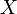$X$ is metrizable and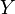$Y$ is homeomorphic to it, a metric on$X$ composed with the homeomorphism from$Y$ to$X$ defines a metric on$Y$ which induces the topology of$Y$. Drorbn 10:00, 25 October 2010 (EDT)
• Question. When proving if X is a metrizable space and A is a countably infinite set in X. The cardinality of the sequential closure of A is less or equal to the cardinality of R we used the fact that a metrizable space is Hausdorff right? Or you can say that the number of limit points should be less or equal to the number of converging sequences... -Kai
• Yes we use the fact that metric spaces are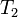$T_2$. Drorbn 20:30, 26 October 2010 (EDT)
• Question. When proving topologist's sine curve is not path connected in page 157. Are we using the fact that for a cts function in Euclidean space if x_n converges to x then f(x_n) has to converge to f(x)? i.e. any open nbd of f(x) contains all but finitely many terms of f(x_n)? -KaiXwbdsb 14:01, 27 October 2010 (EDT)
• We did not complete the proof of that fact in class. There are many ways to complete it, some use what you wrote and some don't. Drorbn 14:48, 27 October 2010 (EDT)
• Question. Is f(t) = (t,t,t...) cts in the R^\omega with uniform topology?.... -Kai Xwbdsb 15:23, 27 October 2010 (EDT)
• Yes. Drorbn 06:58, 28 October 2010 (EDT)

### Solutions

Anne maybe you can help me again to create a page of solution. I tried but I don't know how to do so.

• Kai: page created, content moved. Just edit the navigation to create a new page. Anne.d 21:42, 10 December 2010 (EDT)